summary of site-wide JavaScript functionalityUnited States-English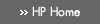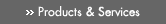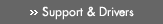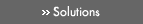» Contact HPSearch:HP Labs All of HP US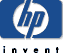# Technical Reports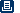printable version»

## HP Labs» Research» News and events » Technical reports» About HP Labs » Careers @ HP Labs » Worldwide sites» DownloadsClick here for full text:Universally Attainable Error-Exponents for Rate- Constrained Denoising of Noisy Sources Weissman, Tsachy HPL-2002-214 Keyword(s): achievable exponents; competitive minimax; compound source; denoising; error exponents; Marton's exponent; Neyman-Pearson; noisy source coding; universal schemes Abstract: Consider the problem of rate-constrained reconstruction of a finite-alphabet discrete memoryless signal X(superscript n) =(X subscript 1), ..., X (subscript n), based on a noise-corrupted observation sequence Z(superscript n), which is the finite-alphabet output of a Discrete Memoryless Channel (DMC) whose input is X(superscript n). Suppose that there is some uncertainty in the source distribution, in the channel characteristics, or in both. Equivalently, suppose that the distribution of the pairs (X(subscript i,), Z(subscript i), rather than completely being known, is only known to belong to a set Theta. Suppose further that the relevant performance criterion is the probability of excess distortion, i.e., letting X(superscript n) (Z(superscript n) denote the reconstruction, we are interested in the behavior of P(subscript theta) rho (X(superscript n), {X}^n(Z(superscript n)) > d theta), where rho is a (normalized) block distortion induced by a single-letter distortion measure and P theta denotes the probability measure corresponding to the case where (X(subscript i), Z (subscript i)) sim theta, theta in Theta. Since typically this probability will either not decay at all or do so at an exponential rate, it is the rate of this decay which we focus on. More concretely, for a given rate R greater than or equal to 0 and a family of distortion levels {d theta} theta in Theta, we are interested in families of exponential levels {I theta} theta in Theta which are achievable in the sense that for large n there exist rate-R schemes satisfying - 1/n log P theta (rho (X(superscript n, X^n (Z(superscript n) ) > d theta greater than or equal to I theta for all theta in Theta. Our main result is a complete ``single- letter'' characterization of achievable levels {I theta} theta in Theta per any given triple (Theta, R, {d theta} theta in Theta. Equipped with this result, we later turn to addressing the question of the ``right'' choice of {I theta} theta in Theta. Relying on methodology recently put forth by Feder and Merhav in the context of the composite hypothesis testing problem, we propose a competitive minimax approach for the choice of these levels and apply our main result for characterizing the associated key quantities. Subsequently, we apply the main result to characterize optimal performance in a Neyman-Pearson-like setting, where there are two possible noise-corrupted signals. In this problem, the goal of the observer of the noisy signal, rather than having to determine which of the two it is (as in the hypothesis testing problem), is to reproduce the underlying clean signal with as high a fidelity as possible (e.g., lowest number of symbol errors when distortion measure is Hamming), under the assumption that one source is active, while operating at a limited information rate R and subject to a constraint on the fidelity of reconstruction when the other source is active. Finally, we apply our result to characterize a sufficient condition for the source class, Theta, to be universally encodable in the sense of the existence of schemes attaining the optimal distribution-dependent exponent, simultaneously for all sources in the class. This condition was shown in an earlier work to suffice for universality in expectation. Notes: This abstract contains mathematical formulae which cannot be represented here. 30 Pages Back to Index## »Technical Reports

» 2009
» 2008
» 2007
» 2006
» 2005
» 2004
» 2003
» 2002
» 2001
» 2000
» 1990 - 1999## Heritage Technical Reports

» Compaq & DEC Technical Reports
» Tandem Technical ReportsPrivacy statement Using this site means you accept its terms Feedback to HP Labs© 2009 Hewlett-Packard Development Company, L.P.